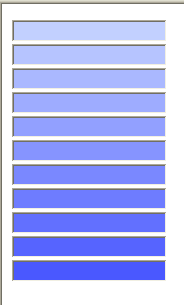# Gradient effect with Web Controls

Applying a different background colour to each textbox or a web control that supports the background attribute can give you an area to play with that can “jazz” up a form/page.

Reference the following namespace

`using System.Collections.Generic;`

Code below

```protected void Page_Load(object sender, EventArgs e)
{
}

public static Control[] GetControlsOnPage(Control root)
{
List<Control> list = new List<Control>();
if (root.HasControls())
{
foreach (Control control in root.Controls)
{

}
}
return list.ToArray();
}

{
Control[] allControls = GetControlsOnPage(Page);
System.Drawing.Color colr = System.Drawing.Color.FromArgb(206, 220, 255);

foreach (Control control in allControls)
{
TextBox textBox = control as TextBox;
if (textBox != null)
{
colr = System.Drawing.Color.FromArgb(colr.R - 12, colr.G - 12, 255);
textBox.BackColor = colr;

}
}
}```

The initial colour is defined on the following line

System.Drawing.Color colr = System.Drawing.Color.FromArgb(206, 220, 255);

The outpage page contains 11 texboxes and the background colours of the boxes are rendered as the following:We could then do something else if it maybe have the colour alternating? We could then change the TextBoxGradient method to.

```  private void TextBoxGradient()
{
Control[] allControls = GetControlsOnPage(Page);
System.Drawing.Color colr = System.Drawing.Color.FromArgb(206, 220, 255);
int i = 0;

foreach (Control control in allControls)
{

TextBox textBox = control as TextBox;
if (textBox != null)
{
i = i +1;
if (i % 2 == 0)
{
colr = System.Drawing.Color.FromArgb(colr.R - 12, colr.G - 12, 255);
textBox.BackColor = colr;
}

}
}
}```

Again using the the same 11 textboxes they are rendered as follows:Another way would be to create several CSS classes that sets each textbox background colour.

Some modifications can be made so that the colour can be applied to border, fore colour or even text colur if the control supports it.

This site uses Akismet to reduce spam. Learn how your comment data is processed.